## Sunday, December 28, 2014

### Topcoder SRM 643 div 2 250 Problem

my approch is
- find H and if there is H find right and find up

 ``` 1 2 3 4 5 6 7 8 9 10 11 12 13 14 15 16 17 18 19 20 21 22 23 24 25 26 27 28 29 30 31 32 33 34 35 36 37 38 39 40 41 42 43 44 45``` ```package srm643; public class TheKingsArmyDiv2 { public static void main(String args[]){ String[] temp = {"SSSSS", "SSHHS", "SSSSS"}; TheKingsArmyDiv2 army = new TheKingsArmyDiv2(); System.out.println(army.getNumber(temp)); } public int getNumber(String[] state){ int len = state.length; int count =0; for(int i=0; i 0){ return 1; }else{ return 2; } } } ```

## Tuesday, December 23, 2014

### Topcoder SRM 640 div2 250point

this question is very easy!!

start and ribons

if left and right is different counting ++

my code is below~

problem
http://community.topcoder.com/stat?c=problem_statement&pm=13556

 ``` 1 2 3 4 5 6 7 8 9 10 11 12 13``` ```public class ChristmasTreeDecorationDiv2 { public int solve(int[] col, int[] x, int[] y){ int len = x.length; int cnt =0; for(int i=0; i

## Monday, December 22, 2014

### SRM 641 div 2 500

this is my source ..

point in triangle is somehow difficult to understand

find the algorithm point in triangle
and when calling pointInTriangle
point.set method called many times.

so later, I have to do refactoring my code to speed up

 ``` 1 2 3 4 5 6 7 8 9 10 11 12 13 14 15 16 17 18 19 20 21 22 23 24 25 26 27 28 29 30 31 32 33 34 35 36 37 38 39 40 41 42 43 44 45 46 47 48 49 50 51 52 53 54 55 56 57 58 59 60 61``` ```package srm641; public class TrianglesContainOriginEasy { public int count(int[] x, int[] y){ int len = x.length; int count = 0; Point v1 = new Point(); Point v2 = new Point(); Point v3 = new Point(); for(int i=0; i

## Sunday, December 21, 2014

### Topcoder SRM 641 250point

 ``` 1 2 3 4 5 6 7 8 9 10 11 12 13 14 15 16 17 18 19 20``` ```public class BuyingTshirts { public int meet(int T, int[] Q, int[] P){ int len = Q.length; int count = 0; int sum = 0; int sum2 = 0; for(int i=0; i= 0 || sum2 -T > 0){ count++; } sum = sum - T; sum2 = sum2 - T ; } return count; } } ```

## Wednesday, December 17, 2014

### Topcoder SRM 642 div2

This is my third time in attending topcoder

and I got 815 grad T_T.

I think my skill and score will be higher than now if I continuously attend and study

http://community.topcoder.com/stat?c=problem_statement&pm=13553&rd=16085&rm=324592&cr=23054155

explanation : first I tried to solve this problem Brute-force Search

It mean all posiible I would search

this is may take O(n) if str length is higer time.

but I tried if str.length is cardinal number ,  find only center and one before, one after

and if str.length . even number search 3point, half and before of half, after of half

ex ) cardinal number : 129298481 : 1292 + 98481,  12929 + 8481

 ``` 1 2 3 4 5 6 7 8 9 10 11 12 13 14 15 16 17 18 19 20 21 22 23 24 25 26``` ```public class ForgetfulAddition { public int minNumber(String str){ int len= str.length() ; int start = len / 2; int minNumber = Integer.MAX_VALUE; if(len % 2 == 1){ // 홀수 int sum = Integer.parseInt(str.substring(0, start)) + Integer.parseInt(str.substring(start, len)); minNumber = Math.min(sum, minNumber); sum = Integer.parseInt(str.substring(0, start+1)) + Integer.parseInt(str.substring(start+1, len)); minNumber = Math.min(sum, minNumber); }else{ // 짝수 int sum = Integer.parseInt(str.substring(0, start)) + Integer.parseInt(str.substring(start, len)); minNumber = Math.min(sum, minNumber); if(len >2){ sum = Integer.parseInt(str.substring(0, start+1)) + Integer.parseInt(str.substring(start+1, len)); minNumber = Math.min(sum, minNumber); sum = Integer.parseInt(str.substring(0, start-1)) + Integer.parseInt(str.substring(start-1, len)); minNumber = Math.min(sum, minNumber); } } return minNumber; } } ```

 ``` 1 2 3 4 5 6 7 8 9 10 11 12 13 14 15 16 17 18 19 20 21 22 23 24 25 26``` ```public class LightSwitchingPuzzle { public int minFlips(String str){ char[] array = str.toCharArray(); int count =0; for(int i=0; i

## Tuesday, December 16, 2014

### Math sqrt in java

sqrt is square root value

Math.sqrt(52) :  7.211102550927978
Math.sqrt(4) : 2
Math.sqrt(9) : 3

Math.floor(1001.56) :  1001
Math.ceil(1001.56) : 1002

## Wednesday, December 3, 2014

### SRM 441 div 1 1000

Recently I solve the problem that was already compteted.
and I found out that I'm not skillfull at understanding english.
I want to understand problem written in english but it is very difficult to get the point of problem,

this is somehow eaiser than others because it show pictures and sample.
I try to solve this problem

next time I hope write answer aticle of this question ~!!!

### Problem Statement

Onise likes to play with paper and paint. He has a piece of paper with dimensions width x height. He performs K operations, one for each i between 0 and K-1, inclusive. Each operation consists of the following steps:
1. Fold the paper along the line x = xfold[i] (the left side of the paper is folded over the right side).
2. Divide the paper vertically into cnt[i]+1 equal sections. Then, cnt[i] times, take the topmost section and fold it over the section below it.
3. Paint a rectangle with the lower-left corner at (x1[i], y1[i]) and the upper-right corner at (x2[i], y2[i]). Note that (0, 0) is now the lower-left corner of the paper in its current folded state, not its original state. The paint will seep through all the layers of the folded paper. See the image below for clarification.
4. Unfold the paper.
For example, let's say Onise has a piece of paper that is 5 x 6. He performs one operation where xfold is 2, cnt is 2, and the coordinates of the painted rectangle's corners are (1, 1) and (3, 2). The following will happen (note that the paper starts out blue in the images and gets painted white):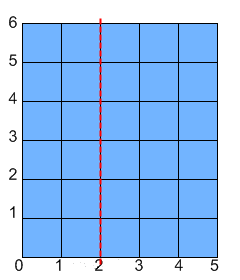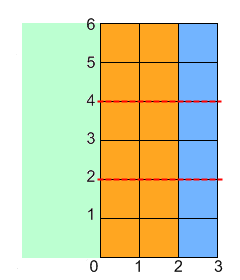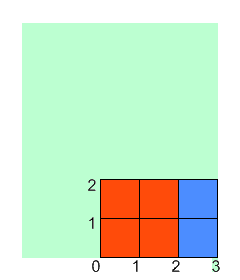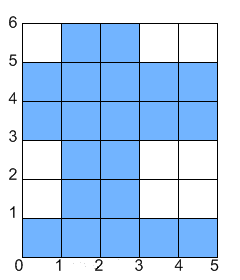You are given ints width and height, and int[]s xfoldcntx1y1x2 and y2, each containing exactly K elements. Return the total area of of the paper that is not covered in paint after Onise is done.

### Definition

 Class: PaperAndPaint Method: computeArea Parameters: int, int, int[], int[], int[], int[], int[], int[] Returns: long Method signature: long computeArea(int width, int height, int[] xfold, int[] cnt, int[] x1, int[] y1, int[] x2, int[] y2) (be sure your method is public)

### Constraints

-width and height will be between 1 and 10^9, inclusive.
-xfoldcntx1y1x2 and y2 will all contain the same number of elements, between 1 and 50, inclusive.
-Every element of xfold will be between 0 and width, inclusive.
-Every element of cnt will be between 0 and 1000, inclusive.
-For every i, cnt[i]+1 will be a divisor of height.
-For every i, 0 <= x1[i] < x2[i] <= max(xfold[i], width-xfold[i]) and 0 <= y1[i] < y2[i] <= height/(cnt[i]+1).

### Examples

0)

 `5` `6` `{2}` `{2}` `{1}` `{1}` `{3}` `{2}`
`Returns: 21`
 The example from the problem statement.
1)

 `2` `4` `{0, 0}` `{1, 0}` `{0, 0}` `{1, 1}` `{2, 1}` `{2, 4}`
`Returns: 3`
 Onise will get the following result:2)

 `6` `6` `{2, 5}` `{1, 2}` `{1, 2}` `{2, 0}` `{3, 4}` `{3, 2}`
`Returns: 18`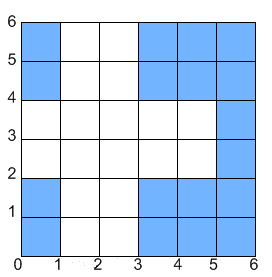3)

 `21` `30` `{3,21,7,11,13}` `{4,14,9,5,4}` `{4,0,2,5,9}` `{2,0,1,2,3}` `{7,19,6,11,12}` `{5,2,2,4,5}`
`Returns: 27`
4)

 `30` `42` `{16, 24, 25, 21, 4}` `{5, 1, 6, 13, 20}` `{3, 1, 5, 8, 9}` `{0, 1, 0, 0, 1}` `{14, 22, 12, 18, 13}` `{2, 15, 1, 1, 2}`
`Returns: 336`
5)

 `26` `60` `{17, 17, 24, 4, 21}` `{4, 1, 11, 0, 2}` `{9, 1, 20, 18, 7}` `{1, 3, 0, 45, 12}` `{13, 4, 23, 19, 13}` `{3, 14, 1, 46, 14}`
`Returns: 1319`
6)

 `17` `3` `{17, 2, 10, 2, 10, 13}` `{2, 0, 0, 2, 0, 0}` `{7, 6, 4, 11, 0, 5}` `{0, 0, 1, 0, 1, 1}` `{12, 10, 6, 12, 4, 12}` `{1, 3, 2, 1, 2, 2}`
`Returns: 20`

`reference by Topcorder`